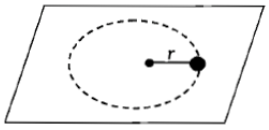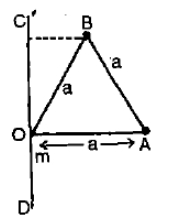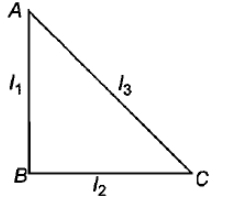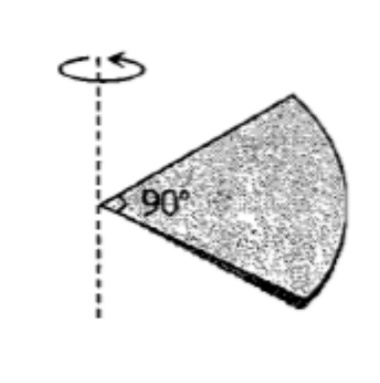A small mass attached to a string rotates on a frictionless table top as shown. If the tension on the string is increased by pulling the string causing the radius of the circular motion to decrease by a factor of 2, the kinetic energy of the mass will1. Increase by a factor of 4

2. Decrease by a factor of 2

3. Remain constant

4. Increase by a factor of 2

Subtopic:  Angular Momentum |
To view explanation, please take trial in the course below.
NEET 2022 - Target Batch - Aryan Raj Singh

Difficulty Level:

Three-point masses 'm' each, are placed at the vertices of an equilateral triangle of side a. Moment of inertia of the system about axis COD is-1. $2m{a}^{2}$

2. $\frac{2}{3}m{a}^{2}$

3. $\frac{5}{4}m{a}^{2}$

4. $\frac{7}{4}m{a}^{2}$

Subtopic:  Moment of Inertia |
To view explanation, please take trial in the course below.
NEET 2022 - Target Batch - Aryan Raj Singh

Difficulty Level:

A particle is moving in a circular orbit with constant speed. Select wrong alternate

1. Its linear momentum is conserved

2. Its angular momentum is conserved

3. It is moving with variable velocity

4. It is moving with variable acceleration

Subtopic:  Linear Momentum |
To view explanation, please take trial in the course below.
NEET 2022 - Target Batch - Aryan Raj Singh

Difficulty Level:

ABC is a right angled triangular plate of uniform thickness. The sides are such that AB > BC as shown in figure.  and ${\mathrm{I}}_{3}$ are moments of inertia about AB, BC and AC respectively. Then, which of the following relation is correct?Subtopic:  Moment of Inertia |
To view explanation, please take trial in the course below.
NEET 2022 - Target Batch - Aryan Raj Singh

Difficulty Level:

One solid sphere A and another hollow sphere B are of same mass and same outer radii. Their moment of inertia about their diameters are respectively IA and IB such that

1. ${\mathrm{I}}_{\mathrm{A}}={\mathrm{I}}_{\mathrm{B}}$

2. ${\mathrm{I}}_{\mathrm{A}}>{\mathrm{I}}_{\mathrm{B}}$

3. ${\mathrm{I}}_{\mathrm{A}}<{\mathrm{I}}_{\mathrm{B}}$

4. $\frac{{\mathrm{I}}_{\mathrm{A}}}{{\mathrm{I}}_{\mathrm{B}}}=\frac{{d}_{A}}{{d}_{B}}$

Subtopic:  Moment of Inertia |
To view explanation, please take trial in the course below.
NEET 2022 - Target Batch - Aryan Raj Singh

Difficulty Level:

A couple produces:                                                               [NTSE 1995; CBSE PMT 1997; DCE 2004]

1. Purely linear motion

2. Purely rotational motion

3. Linear and rotational motion

4. No motion

Subtopic:  Rotation Motion: Introduction |
To view explanation, please take trial in the course below.
NEET 2022 - Target Batch - Aryan Raj Singh

Difficulty Level:

A particle of mass 1 kg is kept at (1m, 1m, 1m). The moment of inertia of this particle about z-axis would be

1.

2.

3.

4.  None of these

Subtopic:  Moment of Inertia |
To view explanation, please take trial in the course below.
NEET 2022 - Target Batch - Aryan Raj Singh

Difficulty Level:

One quarter sector is cut from a uniform circular disc of radius R.  This sector has mass M.  It is made to rotate about a line perpendicular to its plane and passing through the centre of the original disc.  Its moment of inertia about the axis of rotation is [IIT-JEE (Screening) 2001]1. $\frac{1}{2}M{R}^{2}$

2. $\frac{1}{4}M{R}^{2}$

3. $\frac{1}{8}M{R}^{2}$

4. $\sqrt{2}M{R}^{2}$

Subtopic:  Moment of Inertia |
To view explanation, please take trial in the course below.
NEET 2022 - Target Batch - Aryan Raj Singh

Difficulty Level:

A wheel is rotating at the rate of 33 rev/min.  If it comes to stop in 20 s. Then, the angular retardation will be

1. $\mathrm{\pi }\frac{\mathrm{rad}}{{\mathrm{s}}^{2}}$

2.

3.

4.

Subtopic:  Rotation Motion: Introduction |
To view explanation, please take trial in the course below.
NEET 2022 - Target Batch - Aryan Raj Singh

Difficulty Level:

A solid sphere is rotating about a diameter at an angular velocity $\omega$.  If it cools so that its radius reduces to $\frac{1}{n}$ of its  original value, its angular velocity becomes [MP PMT 2006]

1. $\frac{\omega }{n}$                                2. $\frac{\omega }{{n}^{2}}$

3. $n\omega$                               4. ${n}^{2}\omega$

Subtopic:  Angular Momentum |
To view explanation, please take trial in the course below.
NEET 2022 - Target Batch - Aryan Raj Singh

Difficulty Level: